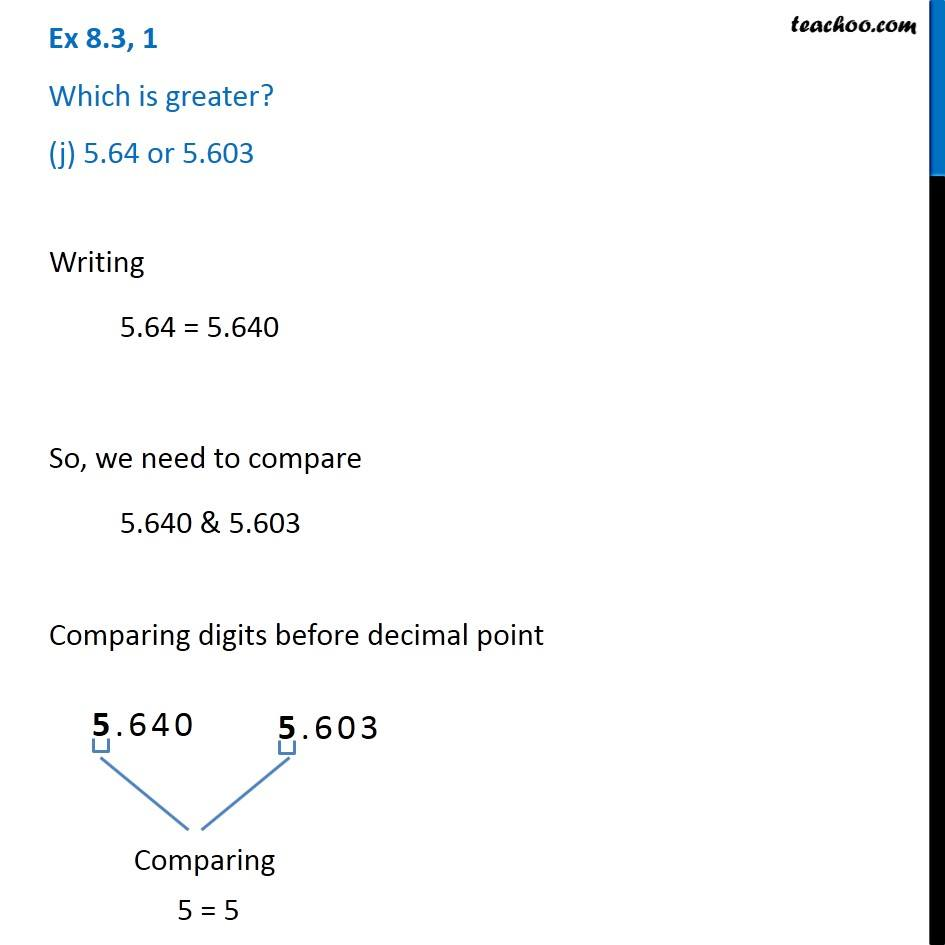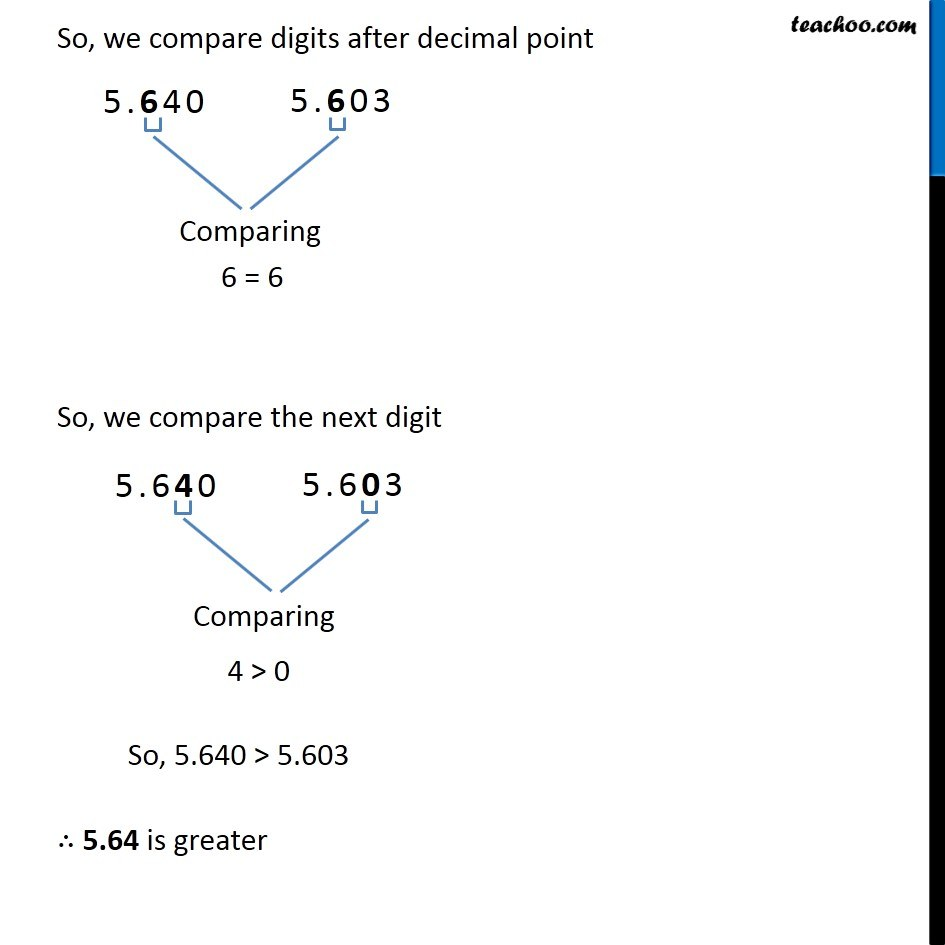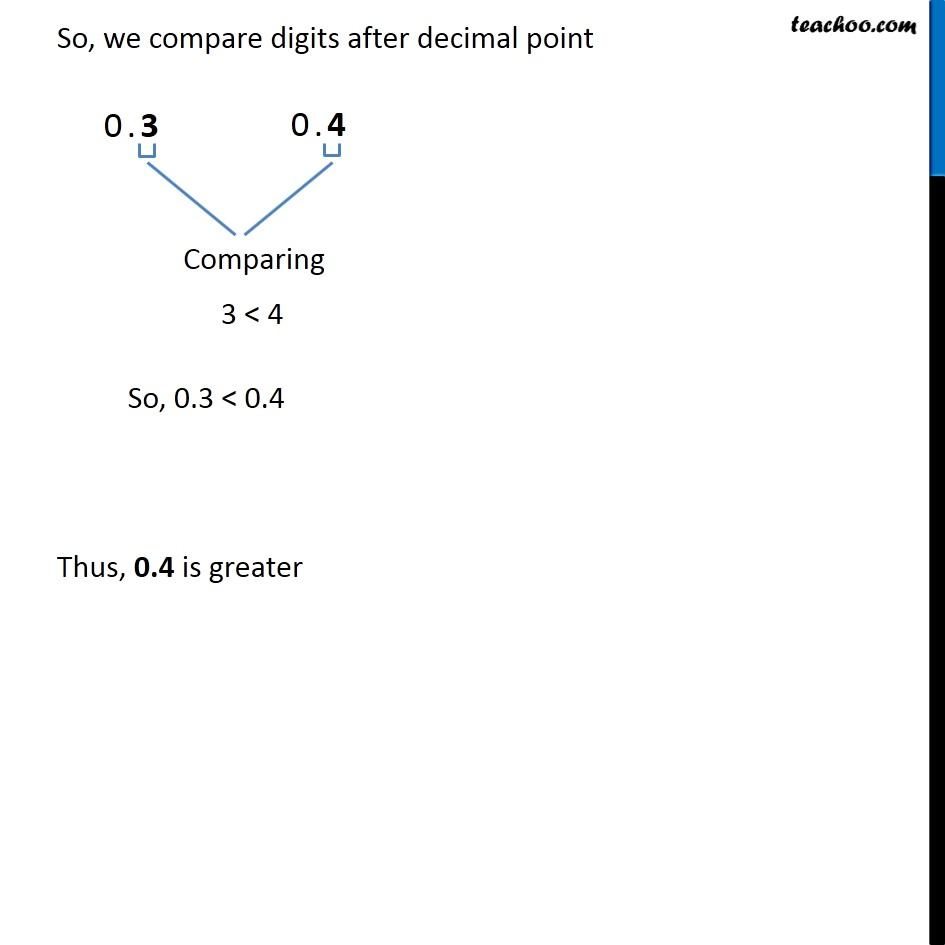Ex 8.3

Chapter 8 Class 6 Decimals
Serial order wise### Transcript

Ex 8.3, 1 Which is greater? (j) 5.64 or 5.603 Writing 5.64 = 5.640 So, we need to compare 5.640 & 5.603 Comparing digits before decimal point 5 = 5 So, we compare digits after decimal point 6 = 6 So, we compare the next digit 4 > 0 So, 5.640 > 5.603 ∴ 5.64 is greater